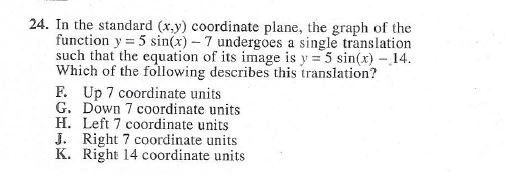Home / Answered Questions / Other / 24-in-the-standard-x-y-coordinate-plane-the-graph-of-the-function-y-5-sin-x-7-undergoes-a-single-tra-aw996

# (Solved): 24. In The Standard (x,y) Coordinate Plane, The Graph Of The Function Y = 5 Sin(x) - 7 Undergoes A S...24. In the standard (x,y) coordinate plane, the graph of the function y = 5 sin(x) - 7 undergoes a single translation such that the equation of its image is y = 5 sin(x) - 14. Which of the following describes this translation? F. Up 7 coordinate units G. Down 7 coordinate units H. Left 7 coordinate units J. Right 7 coordinate units K. Right 14 coordinate units

We have an Answer from Expert